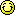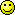### The Logic Forum Discussion AreaLogic
Author
Comment
Philosophy 12A (Logic)

Does anyone know how to solve this problem?

Give a natural deduction proof of the following LMPL sequent. You may use SI and TI (but, you don’t have to).
( ∃x)(F x ↔ Gx), (∀x)[Gx → (Hx → J x)] ⊢ (∃x)J x ∨ [(∀x)F x → (∃x)(Gx & ∼Hx)

Something about you (optional) i am vi

Re: Philosophy 12A (Logic)

Hi. I would recommend you eschew symbols and work with ordinary language. Symbols are just a conceit, designed to make one appear more scientific - but in fact they confuse issues and lead to errors.
Put your problem down on paper in plain English. "for some x, etc....", then order your sentences into syllogism(s). To solve these, resort to diagrams, if necessary.

Something about you (optional) writer in logic, philosophy, spirituality

Re: Philosophy 12A (Logic)

I can get as far as translating the propositions into English, but I don't know how to convert them to syllogisms.

1. For some x, if x is F then x is G, and if x is G then x is F.
2. For all x, if x is G then if x is H then x is J.

=>

3. For some x, either x is J, or for all x, if x is F then for some x, x is G and x is not H

Re: Philosophy 12A (Logic)

Hi Jacobite.
1. For some x, if x is F then x is G, and if x is G then x is F.
2. For all x, if x is G then if x is H then x is J. Whence, ditto for these x (by subsumption from all x).
Therefore, for these (i.e. some) x, if x is F, then x is G (from 1) and maybe also H and thence J (from 2). This implies that these x might eventually be J (the first alternative below). We are not told however whether these x (or any x) may be G and not H; it may well be (given these premises) that for these x, if x is G, then x is necessarily H and thence J.
=> THUS, THE PREMISES 1 & 2 DO NOT NECESSARILY YIELD THE PUTATIVE CONCLUSION 3.

3. Either (For some x, x is J) or (For all x, if x is F then for some x, x is G and x is not H).
The latter alternative = For some x, if x is F then x is G and not H. We are not told whether the some x intended here are the same as the ones intended earlier. We also do not know whether the some x in the first alternative overlap or not with the some x in the second.
WHAT IS EVIDENT HERE IS THAT WHOEVER FORMULATED THIS PROPOSED INFERENCE, ASSUMING THEY THOUGHT THAT THE SAID 2 PREMISES IMPLY THE SAID CONCLUSION, DOES NOT UNDERSTAND THE NATURE OF IMPLICATION… THIS SERVES TO SHOW, TOO, HOW PEOPLE WHO ENGAGE IN SYMBOLIC LOGIC GET CONFUSED AND HIDE OR FAIL TO SEE THEIR ERRORS OF LOGIC.
MOREOVER, JACOBITE, WITH REFERENCE TO OUR PREVIOUS CONVERSATION ABOUT THE FUTILITY OF SYMBOLIC LOGIC, I WOULD LIKE YOU TO NOTE THAT A SENTENCE LIKE “For all x, if x is F then for some x, x is G and x is not H” WOULD NOT OCCUR IN PRACTICE (i.e. in ordinary discourse, though logicians might do that for theoretical purposes). WE DO NOT DRAW PARTICULAR CONSEQUENCES FROM GENERAL CONDITIONS. WE DO NOT SAY: “If all x are F, then some x are G and not H” which is what is being said here.
Please note that I am very busy at this time and cannot respond more than this, unless of course you find an error in my above analysis or anywhere in my work. Thanks.Regarding symbolic logic in general, you make some good points. Logic, after all, is not mathematics.